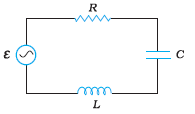Q

# Figure shows a series LCR circuit connected to a variable frequency 230 V source. Show that the potential drop across the LC combination is zero at the resonating frequency.

Q7.11    Figure shows a series LCR circuit connected to a variable frequency  source.

, .

Determine the rms potential drops across the three elements of the circuit. Show that the potential drop across the LC combination is zero at the resonating frequency.Views

Potential difference across any element =

NOW,

The potential difference across capacitor:

Potential difference across the inductor

The potential difference across Resistor

Potential difference across LC combination

Hence at resonating frequency potential difference across LC combination is zero.

Exams
Articles
Questions